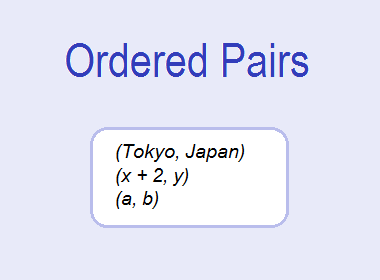# Ordered PairsAn ordered pair is a pair of objects whose components occur in a special (fixed) order. It is written by listing the two components in a specific order, separating them by a comma, and enclosing the pair in parenthesis (small brackets).

Thus, a pair of objects in which order plays an important role is called an ordered pair. We denote the ordered pair made up of x and y by (x, y). We say x is the first element (component) and y is the second element (component) of the ordered pair (x, y).

********************

********************

For example, (Tokyo, Japan) is an ordered pair in which the first component is the capital of the second component. If the component of the ordered pair is interchanged, it will change the sense of the pair also. Hence (Tokyo, Japan) and (Japan, Tokyo) are different ordered pairs.Similarly, the coordinate (2, -3) is an ordered pair in which 2 is the x-coordinate and -3 is the y-coordinate. If the components of the ordered pair are interchanged we get (-3, 2) which is different from (2, -3).

## Equality of Ordered Pairs

Two ordered pairs (a, b) and (c, d) are said to be equal if a = c and b = d. For example, (2, 3) and (6/3, 3) are equal because 2 = 6/3 and 3 = 3. But (2, 5) ≠ (5, 2) because 2 ≠ 5 and 5 ≠ 2.

### Worked Out Example

Example 1: If (x + 2, y) = (5, 4x – 8), find x and y.

Solution: Here,

(x + 2, y) = (5, 4x – 8)

Therefore,

x + 2 = 5 and y = 4x – 8

Now,

x + 2 = 5

or, x = 5 – 2

or, x = 3

Again,

y = 4x – 8

or, y = 4×3 – 8

or, y = 12 – 8

or, y = 4

x = 3 and y = 4 Ans.